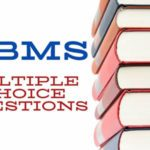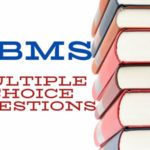# DBMS MCQ Questions and Answer Set-9It Includes the latest DBMS MCQ Questions and Answer.SQL Online test questions and answers. 1.Evaluate the SQL statement: SELECT ROUND (TRUNC (MOD (1600, 10),-1), 2) FROM dual; What will be displayed? a) 0 b) 1 c) C.0.00 d) D.an error statement Ans A 2.Evaluate the SQL statement: TRUNCATE TABLE DEPT; Which three are true about the SQL statement? A. It releases the storage space used by the table. B. It does not release the storage space used by the table. C. Y...

# DBMS MCQ Questions and Answer Set-81. Aggregate functions are functions that take a ___________ as input and return a single value. a) Collection of values b) Single value c) Aggregate value d) Both Collection of values & Single value Answer: a 2. SELECT __________FROM instructor WHERE dept name= ’Comp. Sci.’; Which of the following should be used to find the mean of the salary? a) Mean(salary) b) Avg(salary) c) Sum(salary) d) Count(salary) Answer: b 3. SELECT COUNT (____ ID) FROM teach...

# DBMS MCQ Questions and Answer Set-31. What operator tests the column for the absence of data? A. EXISTS operator B. NOT operator C. IS NULL operator D. None of these Answer:  C 2. In SQL, which command(s) is (are) used to change a table’s storage characteristic? A. ALTER TABLE B. MODIFY TABLE C. CHANGE TABLE D. All of the above Answer:  A 3. A command that lets you change one or more fields in a record is A. Insert B. Modify C. Look-up D. All of the above Answer: B ...